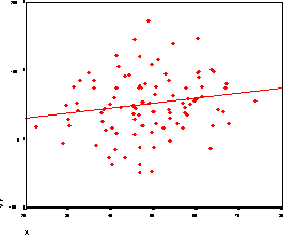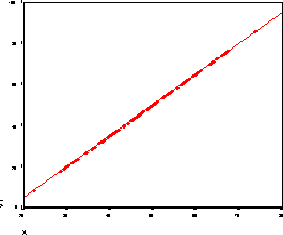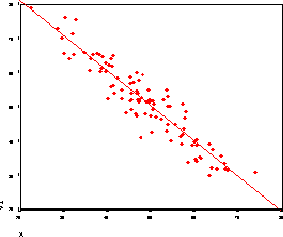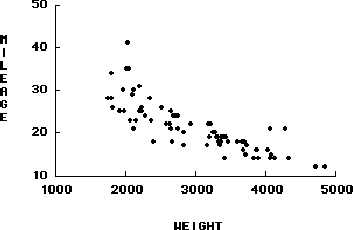# CS533 Sample Final Exam Questions

1. Regression
1. What is the coefficient of determination?

2. For each of the figures below, estimate the correlation coefficient.3. What would you say about the appropriateness of linear regression for the below scatter plot of measured data?2. In measuring the effects of block size on disk read time, an ANOVA test was performed. The variation for the Alternatives (SSA), Error (SSE) and Total (SST) are found to be 2, 8 and 10, respectively. What percent of the total variation is due to differences among alternatives? What percent of the total variation is due to noise in measurements? What can you say overall about the differences among the alternatives?

3. What are some of the reasons that a simulation with more detail can actually be less effective than one with less detail.

4. What is the difference between verification and validation? In performance evaluation using simulation, when do you do validation relative to verification?

5. What are the key differences between emulation, Monte Carlo simulation and discrete-event simulation?

6. What is transient removal? Describe the underlying idea behind most transient removal heuristics. Feel free to provide examples, where appropriate.

7. Determine the average response time of a single-server system in which the mean time between arrivals of jobs is 200 msec and the meant time required to service a job is 50 msec.

8. Show one way it is better, on average, to have one shared queue for each of c servers than it is to have a separate queue for each server.

9. Compare a computer system with a single, powerful process or a multiprocess system with c less powerful processors, each of which can service jobs at 1/c the rate of the single-processor system.Return to the CS533 Home Page